# Find a Side Using the Cosine Rule

In this worksheet, students will apply the cosine rule to find out lengths of missing sides of a non-right-angled triangle.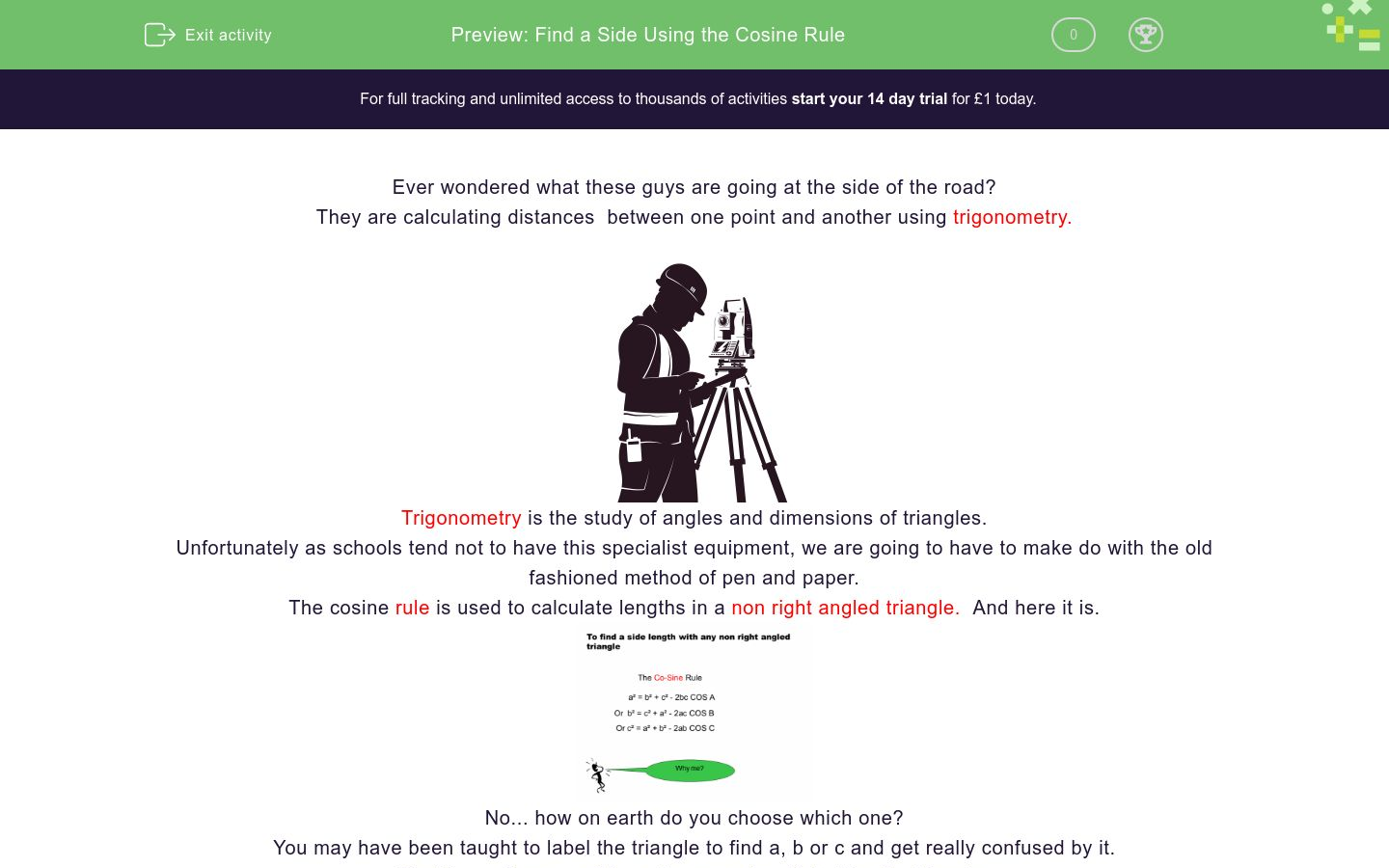Key stage:  KS 4

GCSE Subjects:   Maths

GCSE Boards:   AQA, Eduqas, Pearson Edexcel, OCR

Curriculum topic:   Geometry and Measures, Mensuration

Curriculum subtopic:   Mensuration and Calculation, Triangle Mensuration

Difficulty level:### QUESTION 1 of 10

Ever wondered what these guys are going at the side of the road?

They are calculating distances  between one point and another using trigonometry.Trigonometry is the study of angles and dimensions of triangles.

Unfortunately as schools tend not to have this specialist equipment, we are going to have to make do with the old fashioned method of pen and paper.

The cosine rule is used to calculate lengths in a non right angled triangle.  And here it is.No... how on earth do you choose which one?

You may have been taught to label the triangle to find a, b or c and get really confused by it.

Try this, and you won't have to worry about labelling the triangle.You will need a scientific calculator in order to get the cos button. Make sure it is set to degrees.

Example 1Explanation

We are given two side lengths and the trapped angle.

Square one side, and add the square of the other side.

Subtract 2 x side 1 x side 2 x Cos63(angle)

Example 2Can you see that all you need are the two sides with the trapped angle?

When you use the angle, you will always use the COS button.

As long as you remember the general formula you need not worry about a,b, or c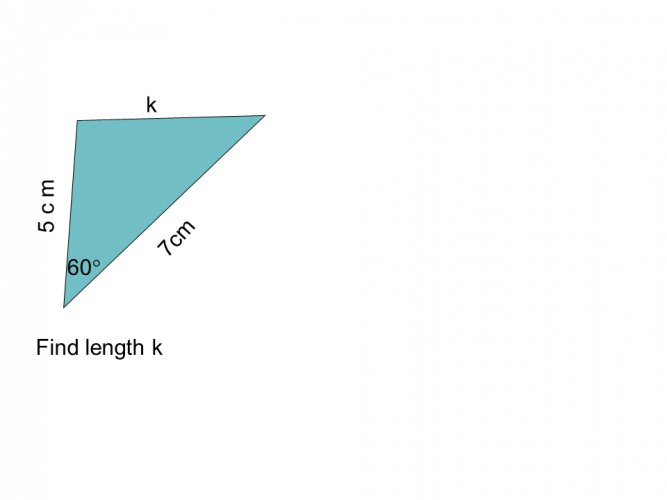Answer has been rounded to 3 significant figures.

20.5 cm

16.1 cm

6.24cm

39.2 cm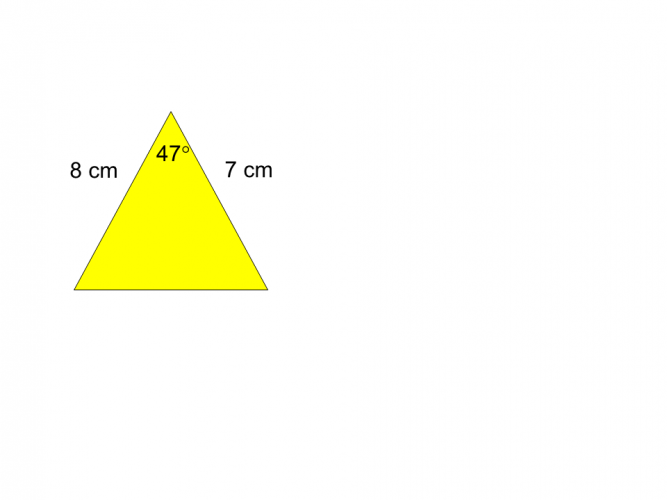7.05 cm 6.50 cm 6.05cm The missing side length =7.05 cm 6.50 cm 6.05cm The missing side length =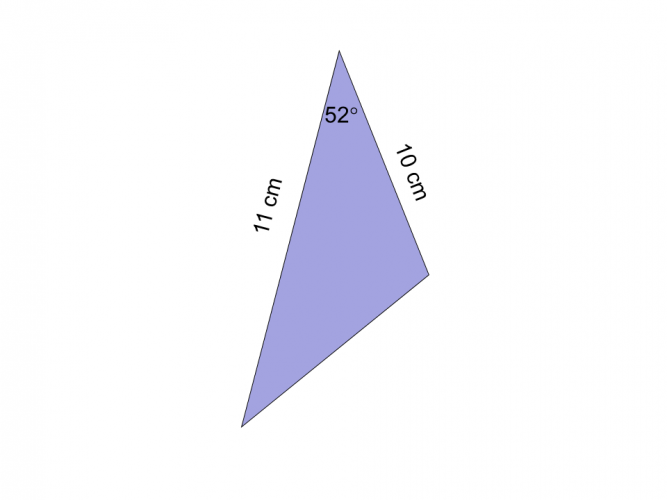Calculate the missing length.What is the length of the missing side?

The answer has been rounded to 3 significant figures (3 s.f)

10.0 cm

10.2cm

10.3 cm

10.1 cm8.95cm 8.92cm 8.91 cm The missing side length is8.95cm 8.92cm 8.91 cm The missing side length isWhat is the length of the missing side.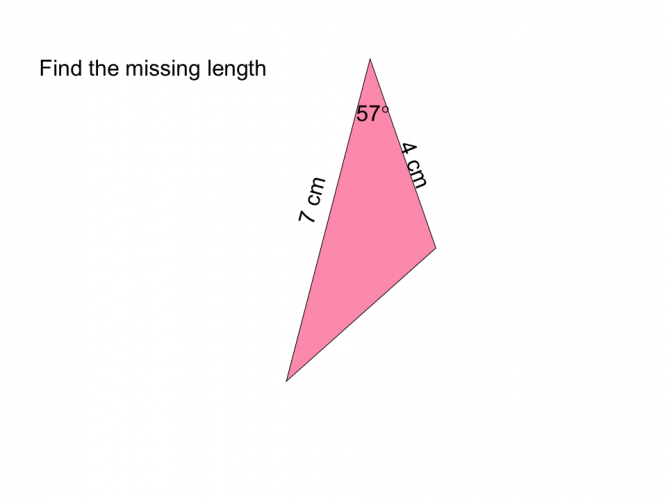14.2 cm

14.5 cm

14.1 cm

14.0 cm

• Question 1Answer has been rounded to 3 significant figures.

6.24cm
EDDIE SAYS
Was it that scary? How did you get on? Did you remember that you need the sides and the trapped angle. 5² + 7² - 2 x 5 x 7 x Cos60 = 39 √39 = 6.24 cm( to 3 s.f) Did you remember the √ ? this is the bit students often forget.
• Question 27.05 cm 6.50 cm 6.05cm The missing side length =
EDDIE SAYS
Can you see the resemblance to Pythagoras theorem? Finding a side length means squaring sides. Once you are comfortable with this it may be that you just need to learn the second part of the formula 8² + 7² - 2 x 7 x 8 x Cos47 = 36.61 √36.61 = 6.05 cm( to 3 s.f)
• Question 3EDDIE SAYS
As there are so many mathematical formulas to remember, this formula is often given to you in text books or at the front of exam papers. As long as you recognise is at using the sides and trapped angle it should take the pressure off you. With practice it soon becomes second nature. 5² + 8² - 2 x 5 x 8 x Cos42 = 29.54 √29.54 = 5.44 cm( to 3 s.f)
• Question 4Calculate the missing length.

9.25
EDDIE SAYS
Is this becoming a little easier now? 10² + 11² - 2 x 10 x 11 x Cos52 = 85.554 √85.554= 9.25 cm( to 3 s.f)
• Question 5What is the length of the missing side?

The answer has been rounded to 3 significant figures (3 s.f)

10.1 cm
EDDIE SAYS
It is always good to write out the formula first and then substitute as it leaves little room for error. Don't try to do this in your head, there is to much information to remember and you could get muddled up. 8² + 5² - 2 x 8 x 5 x Cos100 = 102.98 √102.89= 10.1 cm( to 3 s.f)
• Question 68.95cm 8.92cm 8.91 cm The missing side length is
EDDIE SAYS
Think of using the cosine rule like a triangle. Three things to do 1. Substitute in the numbers 2.Calculate 3. Square root 7² + 4² - 2 x 7 x 4 x Cos105 = 79.493 √79.493 = 8.92cm( to 3 s.f)
• Question 7EDDIE SAYS
Hows it going. Practice, practice, practice is all it takes. That is the beauty of a formula, it gives us step by step instructions. 3² + 3² - 2 x 3 x 3 x Cos42 = 4.623 √4.623= 2.15 cm( to 3 s.f) A good way to check if you are correct is to check that the length is sensible when compared to the other two sides.
• Question 8What is the length of the missing side.

10.3
EDDIE SAYS
8² + 11² - 2 x 8 x 11 x Cos63= 105.09 √105.09= 10.3 cm( to 3 s.f) It is important to be able to round to significant figures as these type of questions often demand it. Yes I know ...something else to practice
• Question 9EDDIE SAYS
Come on admit it, you are having fun now. 7² + 4² - 2 x 7 x 4 x Cos57= 34.500 √34.500 = 5.87 cm( to 3 s.f)
• Question 1014.1 cm
EDDIE SAYS
You are now ready to go and help those surveyors. One last check before you go. 9² + 12² - 2 x 9 x 12 x Cos83 = 198.67 √198.67= 14.1 cm( to 3 s.f)
---- OR ----

Sign up for a £1 trial so you can track and measure your child's progress on this activity.

### What is EdPlace?

We're your National Curriculum aligned online education content provider helping each child succeed in English, maths and science from year 1 to GCSE. With an EdPlace account you’ll be able to track and measure progress, helping each child achieve their best. We build confidence and attainment by personalising each child’s learning at a level that suits them.

Get started